• 主要介绍了Python使用matplotlib和pandas实现的画图操作,结合实例形式分析了Python基于matplotlib和pandas的数值运算与图形显示操作相关实现技巧,并对部分代码的图形显示进行了显示效果测试,需要的朋友可以参考下
• 这里记录一些统计作图方法，包括pandas作图plt作图。前提是先导入第三方库吧import pandas as pd import matplotlib.pyplot as plt import numpy as np然后以下这两句用于正常显示中文标签什么的。plt....
       不得不说使用python库matplotlib绘图确实比较丑，但使用起来还算是比较方便，做自己的小小研究可以使用。这里记录一些统计作图方法，包括pandas作图和plt作图。
前提是先导入第三方库吧
import pandas as pd
import matplotlib.pyplot as plt
import numpy as np
然后以下这两句用于正常显示中文标签什么的。
plt.rcParams['font.sans-serif'] = ['SimHei']  # 用来正常显示中文标签
plt.rcParams['axes.unicode_minus'] = False  # 用来正常显示负号
当然还有一些最基本的步骤:
plt.xticks(x,xtk,size=12,rotation=50) #设置字体大小和字体倾斜度
plt.xlabel(u'城市') # x轴标签
plt.ylabel(u'数量')
plt.title(u'朋友所在城市')  # 图的名称
plt.legend() # 正常显示标题
plt.show()  # 显示图像
plt.close()  # 绘图后养成习惯性的关掉
对于pandas中的二维数据框，可以直接作图（Series类型），简单的折线图或者曲线图如下：
sdata.plot(color='r', style='-o')
plt.show()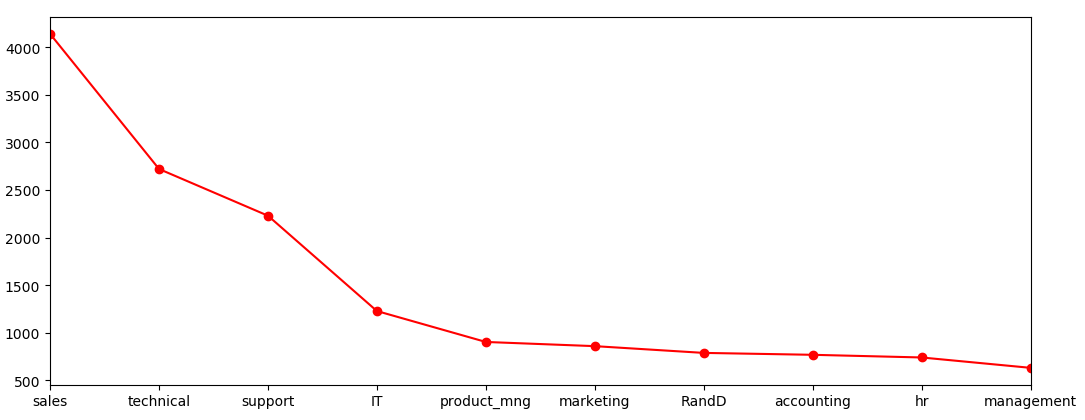如果没有用pandas，直接作曲线图，可以这样写：
plot(x,y, color ='blue', linewidth=2.5, linestyle="--")

1，柱状图
rects1=plt.bar(                      #(x,data) 就是所要画的二维数据
left=x,                      #x 是X坐标轴数据，即每个块的x轴起始位置
height=data,                 #data是Y坐标轴的数据，即每个块的y轴高度
width=[0.1,0.2,0.3],         #每一个块的显示宽度
bottom=[1,2,3],              #每一个块的底部高度
color='y',                   #块的颜色
edgecolor='g',               #块的边界颜色
linewidth=2,                 #块的线条宽度
xerr=1,                      #x轴误差bar
yerr=1,                      #y轴误差bar
ecolor='r',                  #误差bar的颜色
capsize=1,                   #误差bar的线条宽度
orientation='vertical',     #块的方向  (horizontal,vertical)
align="center",              #块的位置 (center, left, right)
hold=None
)

plt.show()

2，饼图
plot2=plt.pie(data,                          # 每个饼块的实际数据，如果大于1，会进行归一化，计算percentage
explode=[0.0,0.1,0.2],               # 每个饼块离中心的距离
colors=['y','r','g'],               # 每个饼块的颜色
labels=['women','men','unknown'],   # 每个饼块的标签
labeldistance=1.2,                   # 每个饼块标签到中心的距离
autopct='%1.1f%%',                  # 百分比的显示格式
pctdistance=0.4,                     # 百分比到中心的距离
startangle=0,                        # 默认从x轴正半轴逆时针起
)
plt.axis('equal')  # 显示为圆形，避免比例压缩为椭圆
plt.show()
3，共享X轴，Y轴左右轴标（帕累托分析图）
数据样例如下，名称为va，类型为Series，左边为职位名称，右边为数量：
sales          4140
technical      2720
support        2229
IT             1227
product_mng     902
marketing       858
RandD           787
accounting      767
hr              739
management      630作图：

fr = pd.Series(va.values.cumsum() / va.values.sum())
va.plot(kind='bar')
fr.plot(color='r',secondary_y=True, style='-o')
plt.annotate(format(fr, '.2%'), xy=(7, fr), xytext=(7*0.9, fr*0.9),
# plt.rcParams['font.sans-serif'] = ['SimHei']  # 用来正常显示中文标签
# plt.rcParams['axes.unicode_minus'] = False  # 用来正常显示负号
# plt1 = plt.pie(va.values,
#                labels=va.index,
#                autopct='%1.1f%%')
plt.xticks(rotation=50)  # 设置字体大小和字体倾斜度
plt.show()
左边为出现的频率，右边为累积百分比（这里数据以降序排列较好，便于直观地观察），效果如下：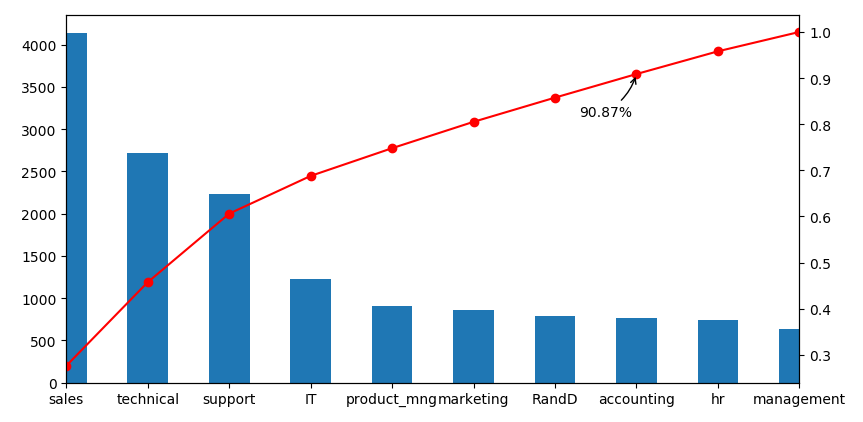在pandas中，曲线图可以直接画，比如data中有多个属性，可以直接使用data.plot()。使用plt，若各个属性需要共用XY轴，那么可以重复plot即可。
4，箱型图
使用pandas画箱型图简单方便，但是注释比较麻烦，可以用annotate添加异常点的注释。若使用之前的数据va，则先创建二维数据框再画图。如果有多个列为数字类型，那么可以画每个列的箱型图，这里只有一列数据，如下：
pd.DataFrame(va).boxplot()
plt.show()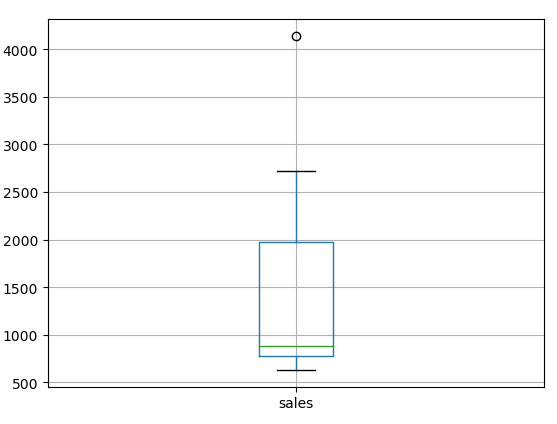使用plt直接进行作图：
plt.boxplot(data,labels=[]，
sym='o',whis=1.5)
其中，data可以是一维的，也可多维，若为多维则lables为每一维度的标签。sym为异常值的形状，whis为调节垂直线段的长度。效果如下：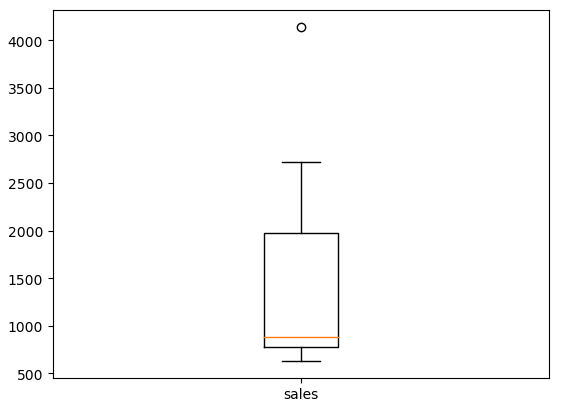5，多张图在一张画布中，即多个子图
使用plt:
plt.subplot(221)
plt.plot(x, y1,'r-', lw=2)
plt.subplot(222)
plt.plot(x,y2)
使用pandas：
data.plot(subplots=True, color=['r','b'], style=['-o','-'])
plt.show()

排版方式有不同，pandas是垂直排列，plt可以自己指定位置。pandas效果如下：展开全文python基本作图
•  为了方便科学计算，Numpy库定义了一些属性方法以便于对一维数据，二位数据高维数据的处理。为了满足科学计算的需求，Numpy定义了一个多维数组对象——ndarray。Ndarray由实际数据描述这些数据的元数据（如...
数据分析整理——嵩天老师mooc笔记
一、Numpy库
为了方便科学计算，Numpy库定义了一些属性和方法以便于对一维数据，二位数据和高维数据的处理。为了满足科学计算的需求，Numpy定义了一个多维数组对象——ndarray。Ndarray由实际数据和描述这些数据的元数据（如数据维度、数据类型）构成，ndarray一般要求所有元素类型相同。
(1)   Ndarray中的属性
属性  说明  .dim  秩，即轴的数量或数据的维度  .shape  ndarray对象的尺度，对应于矩阵的n行m列  .size  ndarray对象中元素的个数，即n*m  .dtype  ndarray对象中元素的类型  .itemsize  ndarray对象中每个元素的大小
ndarray中数据类型包括：bool，intc，intp，int8，int16，int32，int64，uint8，uint16，uint32，uint64，float6，float32，float64，complex64，complex128。
(2)   Ndarray对象的创建
Ndarray对象可以由python中的列表和元组对象创建、使用ndarray中的函数进行创建、从字节流中创建和从文件中读取特定格式进行创建。
l  使用python中的列表和元组对象进行创建
基本格式为 X = np.array（list/tuple，dtype = 数据类型）dtype参数如果没有指定，那么ndaary会根据数据形式自动定义数据类型
l  使用ndarray中的创建函数
函数  说明  np.arange(n)  类似range()函数，返回ndarray类型，元素从0到n‐1  np.ones(shape)  根据shape生成一个全1数组，shape是元组类型  np.zeros(shape)  根据shape生成一个全0数组，shape是元组类型  np.full(shape,val)  根据shape生成一个数组，每个元素值都是val  np.eye(n)  创建一个正方的n*n单位矩阵，对角线为1，其余为0  np.ones_like(a)  根据数组a的形状生成一个全1数组  np.zeros_like(a)  根据数组a的形状生成一个全0数组  np.full_like(a,val)  根据数组a的形状生成一个数组，每个元素值都是val
(3)   Ndarray对象的变换
Ndarray对象的变换是指对ndarray对象的维度和元素类型进行变换。
方法  说明  .reshape(shape)  不改变数组元素，返回一个shape形状的数组，原数组不变  .resize(shape)  与.reshape()功能一致，但修改原数组  .swapaxes(ax1,ax2)  将数组n个维度中两个维度进行调换  .flatten()  对数组进行降维，返回折叠后的一维数组，原数组不变  .astype(new_type)  创建新的数组（原始数据的一个拷贝），但是数据类型进行改变  .tolist()  ndarray数组向列表的变化
(4)   数组的索引和切片

一维数组的索引和切片与python中的列表操作相同。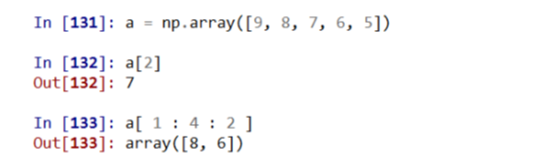多维数组的索引和切片中不同维度的索引序号间要加上逗号进行分隔。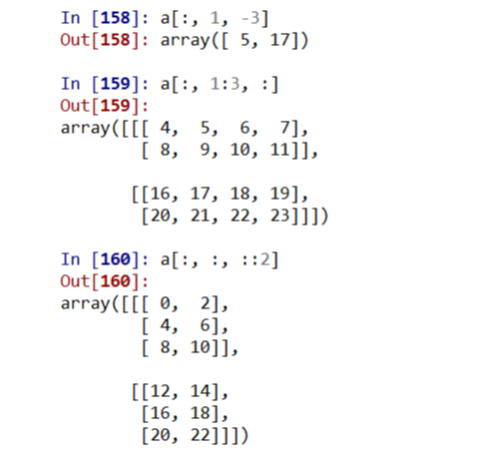(5)   Ndarray数组的运算
函数  说明  np.abs(x) np.fabs(x)  计算数组各元素的绝对值  np.sqrt(x)  计算数组各元素的平方根  np.square(x)  计算数组各元素的平方  np.log(x) np.log10(x) np.log2(x)  计算数组各元素的自然对数、10底对数和2底对数  np.rint(x)  计算数组各元素的四舍五入值  np.modf(x)  将数组各元素的小数和整数部分以两个独立数组形式返回  np.exp(x)  计算数组各元素的指数值  np.sign(x)  计算数组各元素的符号值，1(+), 0, ‐1(‐)        np.cos(x) np.cosh(x) np.sin(x) np.sinh(x) np.tan(x) np.tanh(x)  计算数组各元素的普通型和双曲型三角函数  + ‐ * / **  两个数组各元素进行对应运算  np.maximum(x,y) np.fmax() np.minimum(x,y) np.fmin()  元素级的最大值/最小值计算  np.mod(x,y)  元素级的模运算  np.copysign(x,y)  将数组y中各元素值的符号赋值给数组x对应元素
(6)   数组的读取和写入
函数  说明  a.tofile(frame, sep='', format='%s')  存取函数，frame是写入的文件名，seq是指定的分隔符，format是写入的格式  np.fromfile(frame,dtype=float,count=‐1, sep='')  读取函数，frame是读取的文件名，count代表读取的元素个数，-1表示读取整个文件。注意该方法需要知道数组的维度信息  np.save(fname, array)  Frame是文件名，以npy为拓展名，array是数组变量  np.load(frame)
(7)   随机数函数
函数  说明  rand(d0,d1,..,dn)  根据d0‐dn（维度信息）创建随机数数组，浮点数，[0,1)，均匀分布  randn(d0,d1,..,dn)  根据d0‐dn创建随机数数组，标准正态分布  randint(low,high,shape)  根据shape创建随机整数或整数数组，范围是[low, high)  seed(s)  随机数种子，s是给定的种子值  shuffle(a)  根据数组a的第1轴进行随排列，改变数组x  permutation(a)  根据数组a的第1轴产生一个新的乱序数组，不改变数组x  choice(a[,size,replace,p])  从一维数组a中以概率p抽取元素，形成size形状新数组 replace表示是否可以重用元素，默认为False  uniform(low,high,size)  产生具有均匀分布的数组,low起始值,high结束值,size形状  normal(loc,scale,size)  产生具有正态分布的数组,loc均值,scale标准差,size形状  poisson(lam,size)  产生具有泊松分布的数组,lam随机事件发生率,size形状
(8)   统计函数
函数  说明  sum(a, axis=None)  根据给定轴axis计算数组a相关元素之和，axis整数或元组  mean(a, axis=None)  根据给定轴axis计算数组a相关元素的期望，axis整数或元组  average(a,axis=None,weights=None)  根据给定轴axis计算数组a相关元素的加权平均值  std(a, axis=None)  根据给定轴axis计算数组a相关元素的标准差  var(a, axis=None)  根据给定轴axis计算数组a相关元素的方差  min(a) max(a)  计算数组a中元素的最小值、最大值  argmin(a) argmax(a)  计算数组a中元素最小值、最大值的降一维后下标  unravel_index(index, shape)  根据shape将一维下标index转换成多维下标  ptp(a)  计算数组a中元素最大值与最小值的差  median(a)  计算数组a中元素的中位数（中值）  np.gradient(f)  计算数组f中元素的梯度，当f为多维时，返回每个维度梯度
二、Matplotlib库
Matplotlib库由各种可视化类构成，内部结构复杂，受Matlab启发matplotlib.pyplot是绘制各类可视化图形的命令子库，相当于快捷方式。
(1)   pyplot.plot函数
函数基本格式为plt.plot(x, y, format_string, **kwargs)。x为x轴的数据，y为y周的数据，format_string是控制曲线的格式字符串，包括颜色字符，风格字符和标记字符。
颜色字符  说明  颜色字符  说明  ‘b’  蓝色  ‘m’  洋红色  ‘g’  绿色  ‘y’  黄色  ‘r’  和红色  ‘k’  黑色  ‘c’  青绿色  ‘w’  白色  ‘#008000’  RGB某种颜色  ‘0.8’  灰度值字符串

风格字符  说明  ‘-’  实线  ‘--’  破折线  ‘-.’  点划线  ‘:’  虚线  '' ' '  无线条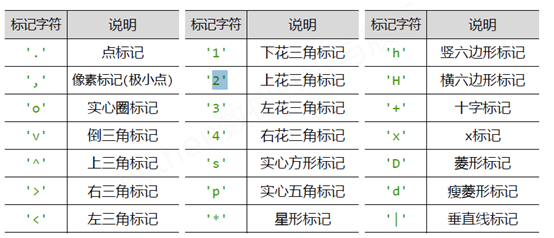pyplot并不默认支持中文显示，需要rcParams修改字体实现。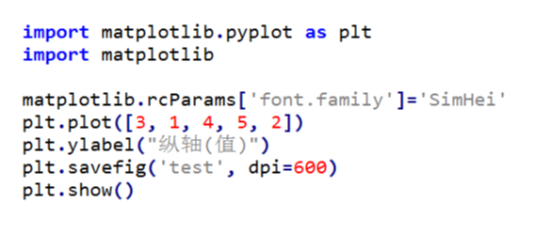rcParams中的参数：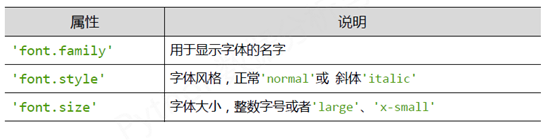除了上述方法外，还可以在有中文输出的地方，增加一个属性：fontproperties。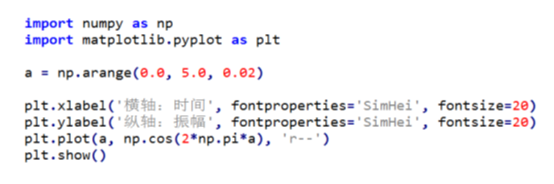文本显示函数：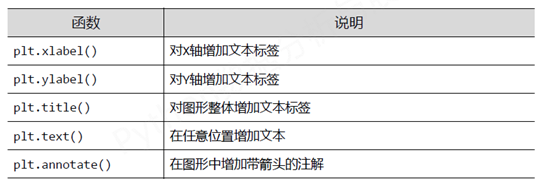(2)   pyplot的子绘图区域
可以使用subplotgrid函数，函数的一般形式为：
plt.subplot2grid(GridSpec, CurSpec, colspan=1,rowspan=1)
colspan是指列上的延伸范围，同理rowspan是行方向的延伸范围。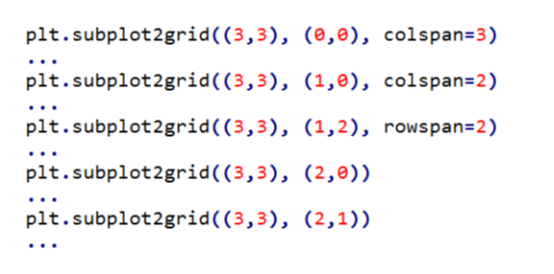此外还可以使用GridSpec类。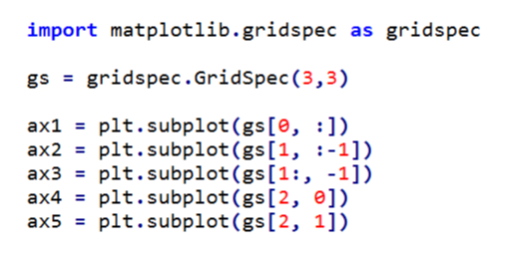图只是展示数据的一种方式，图像的绘制要结合数据特征来看，而且也比较简单，有了数据知道该如何展示后去matplotlib官网上找相应的代码就好了。
(3)   图像的数组展示和处理
图像一般使用RGB色彩模式，即每个像素点的颜色由红(R)、绿(G)、蓝(B)组成，三个颜色通道的变化和叠加得到各种颜色，每个颜色通道的变化范围为0-255。图像是一个由像素组成的二维矩阵，每个元素是一个RGB值，即shape为(lenth，width，3)。
PIL库是一个具有强大图像处理能力的第三方库，其中定义了Image类，可以使图像变成数组，并对其进行处理，最后再把数组返回成图像。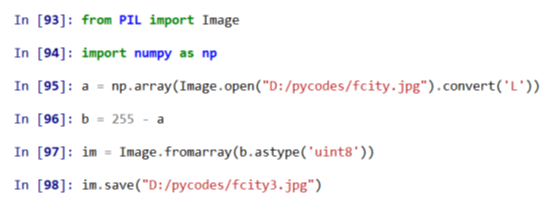convert(‘L’)表示把图像转化成灰度值。
三、Pandas库
Pandas是Python第三方库，提供高性能易用数据类型和分析工具，其定义了两个数据类型：Series，DataFrame。不同于numpy库中定义的ndarray类型，pandas库中的数据类型关注数据的应用，即数据和索引之间的关系。
(1)   Series类型
Series类型由一组数据及与之相关的数据索引组成，只用于表达一维数组。Series类型可以由python列表和字典、ndarray和其他函数创建。
创建的一般表达为，pd.Series(array，index=)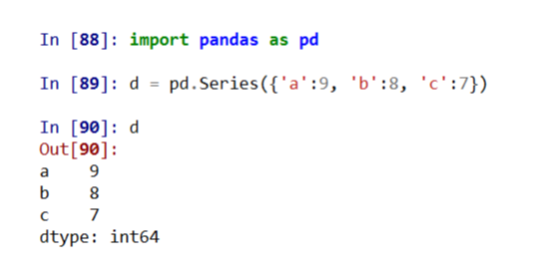Series类型的操作类似ndarray类型：索引方法相同，采用[]、NumPy中运算和操作可用于Series类型、可以通过自定义索引的列表进行切片以及可以通过自动索引进行切片，如果存在自定义索引，则一同被切片。
Series类型的操作类似Python字典类型：通过自定义索引访问、保留字in操作以及使用.get()方法。
(2)   DataFrame类型
DataFrame类型由共用相同索引的一组列组成。DataFrame是一个表格型的数据类型，每列值类型可以不同DataFrame既有行索引、也有列索引DataFrame常用于表达二维数据，但可以表达多维数据。操作和床架与Series类型相似。
(3)   索引类型的函数

方法  说明  .reindex(index=None,columns=None..)  改变或重排Series和DataFrame索引  .append(idx)  连接另一个Index对象，产生新的Index对象  .diff(idx)  计算差集，产生新的Index对象  .intersection(idx)  计算交集  .union(idx)  计算并集  .delete(loc)  删除loc位置处的元素  .insert(loc,e)  在loc位置增加一个元素e  .drop()  删除Series和DataFrame指定行或列索引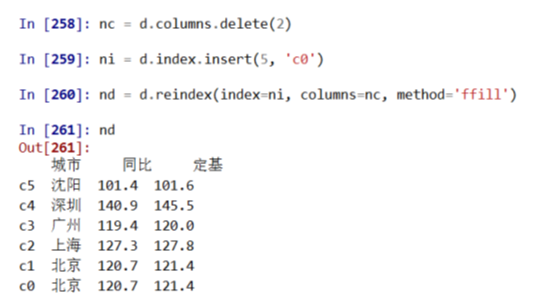(4)   数据类型运算
算术运算根据行列索引，补齐后运算，运算默认产生浮点数，补齐时缺项填充NaN (空值)。二维和一维、一维和零维间为广播运算。
方法  说明  .add(d, **argws)  类型间加法运算，可选参数  .sub(d, **argws)  类型间减法运算，可选参数  .mul(d, **argws)  类型间乘法运算，可选参数  .div(d, **argws)  类型间除法运算，可选参数
上述函数的axis参数默认为0，即在行方向上进行运算，改为1则在列的方向上进行运算。
(5)   统计运算
方法  说明  .sort_values(by,axis=0,ascending=True)  在指定轴上根据数值进行排序，默认升序  .sum()  计算数据的总和，按0轴计算，下同  .count()  非NaN值的数量  .mean() .median()  计算数据的算术平均值、算术中位数  .var() .std()  计算数据的方差、标准差  .min() .max()  计算数据的最小值、最大值  .argmin() .argmax()  计算数据最大值、最小值所在位置的索引位置（自动索引）  .idxmin() .idxmax()  计算数据最大值、最小值所在位置的索引（自定义索引）  .describe()  针对0轴（各列）的统计汇总  .rolling(w).sum()  依次计算相邻w个元素的和  .cov()  计算协方差矩阵  .corr()  计算相关系数矩阵, Pearson、Spearman、Kendall等系数 
展开全文python numpy
• 使用sk-learn，numpy，matplotlib和pandas进行通用机器学习的python框架 帮助您准备数据，训练保存模型，处理不平衡问题，绘制决策余量，将树保存到文件中，为您提供功能重要性最重要的标签。 验证精度，召回率...
• JoyPy是一个基于matplotlib +熊猫的单功能Python软件包，目的是：绘制Joyplots（又称山脊线图）。 JoyPy的代码借鉴了pandas.plotting中的pandas.plotting ，并在其中使用了一些实用程序函数。 什么是摇杆？ ...
• matplotlib和pandas实现多y轴的多图形绘制 我们知道pandas支持次Y轴，但是将三次Y轴或者多Y轴放置在绘图上的方法应该是怎么样的呢。效果图与代码实现如下。 import pandas as pd import numpy as np import ...


matplotlib和pandas实现多y轴的多图形绘制

我们知道pandas支持次Y轴，但是将三次Y轴或者多Y轴放置在绘图上的方法应该是怎么样的呢。效果图与代码实现如下。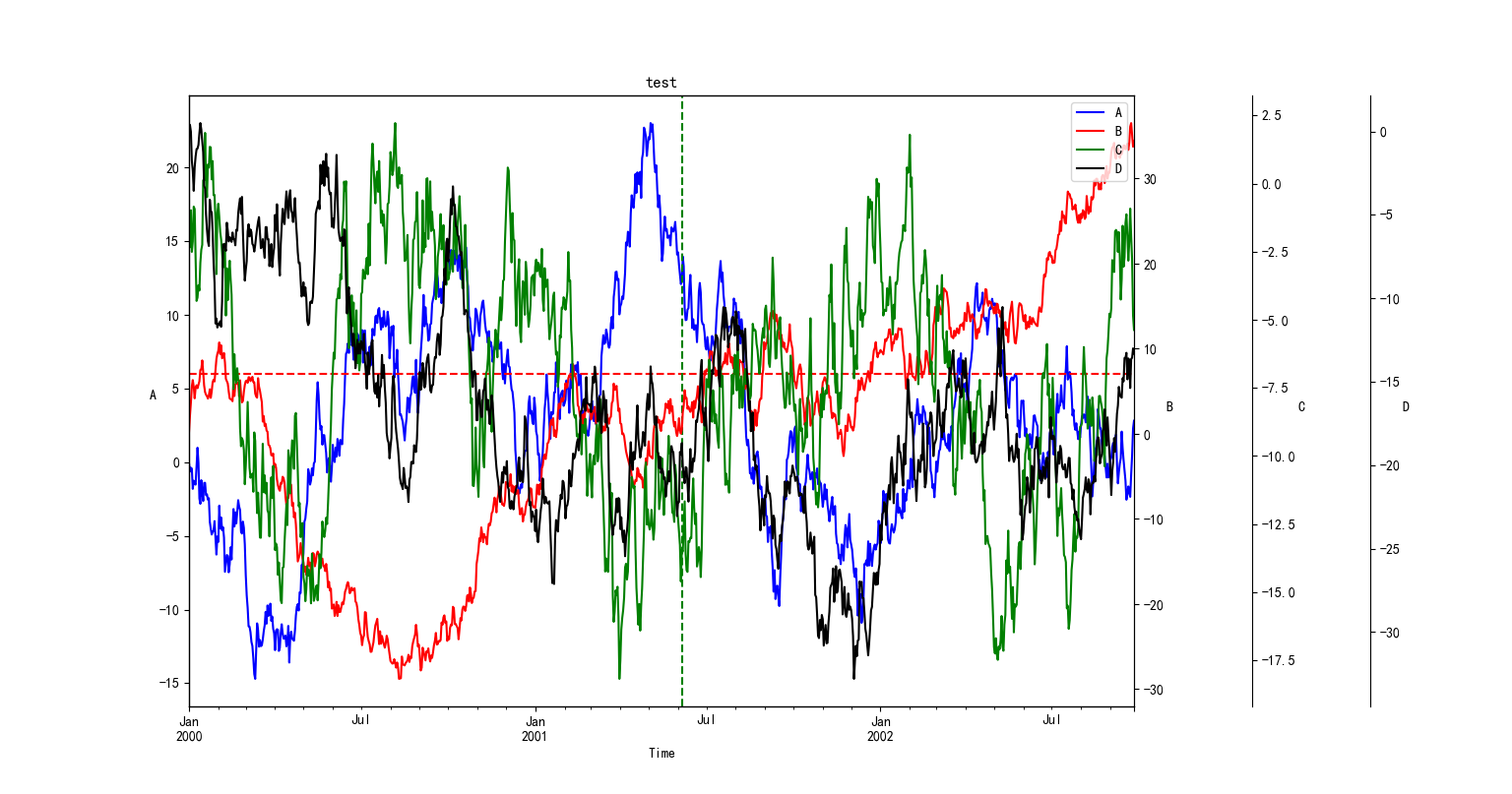import pandas as pd
import numpy as np
import matplotlib.pyplot as plt

ts = pd.Series(np.random.randn(1000),index=pd.date_range('1/1/2000', periods=1000))
ts = ts.cumsum()
df =pd.DataFrame(np.random.randn(1000, 4), index=ts.index, columns=list('ABCD'))
df = df.cumsum()

fig, ax = plt.subplots()
ax2, ax3 ,ax4 = ax.twinx(), ax.twinx() ,ax.twinx()
rspine = ax3.spines['right']
rspine.set_position(('axes', 1.125))
# ax3.set_frame_on(True)
ax3.patch.set_visible(False)
rspine = ax4.spines['right']
rspine.set_position(('axes', 1.25))
ax4.patch.set_visible(False)

df.A.plot(ax=ax, style='b-',legend=False)
df.B.plot(ax=ax2,style='r-',legend=False)
df.C.plot(ax=ax3, style='g-',legend=False)
df.D.plot(ax=ax4, style='k-',legend=False)

#设置图例，如果没有这行代码会使图例重叠
ax4.legend([ax.get_lines(), ax2.get_lines(), ax3.get_lines(),ax4.get_lines()], ['A', 'B', 'C','D'],loc='best')

ax.set_xlabel("Time")
ax.set_ylabel('A',rotation=0)
ax2.set_ylabel('B',rotation=0)
ax3.set_ylabel('C',rotation=0)
ax4.set_ylabel('D',rotation=0)
ax.axhline(6, color="red", linestyle="--")
ax.axvline("2001-06-05", color="green", linestyle="--")

plt.title("test")

# 加这个两句 可以显示中文
plt.rcParams['font.sans-serif'] = [u'SimHei']
plt.rcParams['axes.unicode_minus'] = False

plt.show()

展开全文python
• 使用库Numpy，Matplotlib和Pandas进行股票市场数据集探索性数据分析的Jupyter Notebook。 数据集具有以下功能： serial_number：每个变量的唯一标识符 time_stamp：捕获数据的日期 open_value：指定日期的期初值 ...
• 足球分析仪 利用matplotlib和Pandas比较不同足球运动员球队之间统计数据的Python Web应用程序。SCSS
• Matplotlib库绘图 Matplotlib是一个非常好用的高质量绘图工具库，可以根据输入的数据绘制各种图形，如折线图、柱状图、饼图、散点图等。...准备绘图时要用到的数据，xy表示与x轴y轴对应的数据。 >
用Matplotlib库绘图
Matplotlib是一个非常好用的高质量绘图工具库，可以根据输入的数据绘制各种图形，如折线图、柱状图、饼图、散点图等。
1．绘制折线图
折线图是用直线段将各数据点连接起来而组成的图形，以折线方式显示数据的变化趋势。下面我们绘制一个简单的折线图。
从Matplotlib库导入pyplot模块，简称plt。
>>> import matplotlib.pyplot as plt
准备绘图时要用到的数据，x和y表示与x轴和y轴对应的数据。
>>> x=[0,1,2,3,4,5]
>>> y=[0,1,4,9,16,25]
调用模块的plot函数绘图。
>>> plt.plot(x,y)
调用模块的savefig函数把图形保存为矢量图。
>>> plt.savefig('H:\示例\第9章\plt_line.svg')
用浏览器打开矢量图，效果如图9-10所示。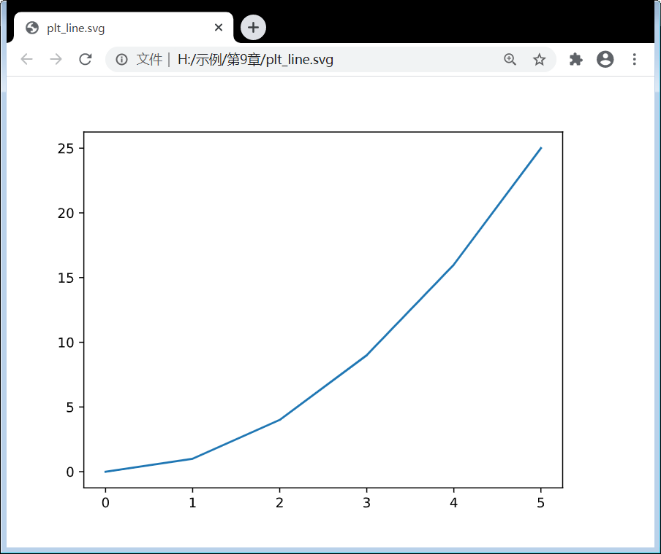图9-10
plot函数包括许多参数，除了数据之外，常用的参数如下：color表示折线的颜色，marker表示折线上数据点处的标记风格，linestyle表示折线的类型，linewidth表示线条粗细，markersize表示数据点标记大小。
>>> plt.plot(x,y,color='red', marker='o', linestyle='dashed', linewidth=1, markersize=5)
调用模块的show函数显示图形到屏幕。
>>> plt.show()
显示效果如图9-11所示。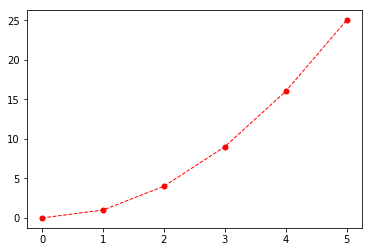图9-11
颜色（color）、标记风格（marker）、线条样式（linestyle）的设置可以进一步简写，可以用每个属性的缩写。例如，“ro:”表示红色、圆点、虚线。
>>> plt.plot(x,y,'ro:', linewidth=1, markersize=5)
常用的颜色缩写包括：蓝（b）、绿（g）、红（r）、蓝绿（c）、洋红（m）、黄（y）、黑（k）、白（w）。
常用的点型缩写包括：点标记（.）、像素标记（,）、实心圈标记（o）、倒三角标记（v）、上三角标记（^）、左三角标记（<）、右三角标记（>）、下花三角标记（1）、上花三角标记（2）、左花三角标记（3）、右花三角标记（4）、实心方形标记（s）、五边形标记（p）、星标记（*）、竖六边形标记（h）、横六边形标记（H）、加号标记（+）、叉标记（x）、钻石标记（D）、菱形标记（d）、竖线标记（|）、横线标记（_）。
常用的线型缩写包括：实线（-）、破折（--）、点画线（-.）、虚线（:）、无线条（空格）。
在Matplotlib中画图，要提供x轴所有的数值以及y轴所有的数值，而且这两个数值列表的数目要能够逐一配对，也就是一个x值要搭配一个y值。上例中，实际上就是将（0,0）、（1,1）、（2,4）、（3,9）、（4,16）、（5,25）这6个点连接起来。
要注意的是，当只输入一维数据的时候，自动将其当作y轴数值处理，x轴默认生成[0,1,2,…]。
下面我们在图中绘制多条折线图。
   import matplotlib.pyplot as plt
x=[0,1,2,3]
y0=[4,6,8,10]
y1=[4,9,16,25]
⓿ plt.plot(x,y0,'bo--',label='电脑')
plt.plot(x,y1,'rp-',label='手机')
❶ plt.legend(loc='best')
❷ plt.rcParams['font.sans-serif']=['SimHei']
❸ plt.title('2019年各产品销量(单位：万台)', fontsize=20)
index_name=['1季度', '2季度', '3季度', '4季度']
❹ plt.xticks(x, index_name)
plt.show()
语句⓿绘制两条不同风格的折线；语句❶调用legend函数设置图例位置；语句❷调用rcParams函数设置中文字体；语句❸调用title函数设置图表标题；语句❹调用xticks函数设置x轴数值，最后调用show函数显示图形，效果如图9-12所示。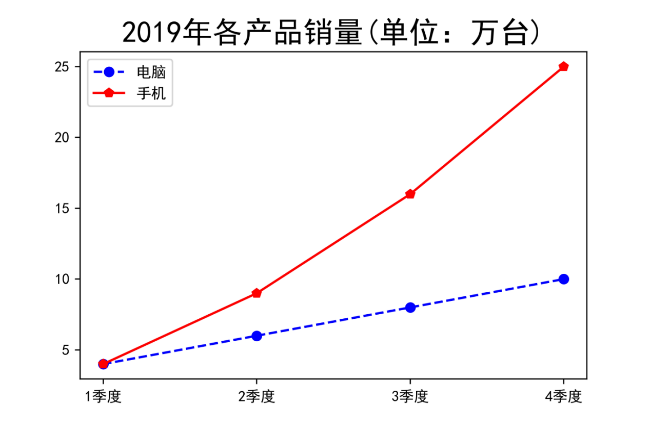图9-12
2．绘制其他类型图形
折线图是基于plt.plot函数绘制的。Matplotlib库还提供了许多不同种类图形的函数。

下面我们将多种类型的图形绘制在一起。

   import matplotlib.pyplot as plt
plt.rcParams['font.sans-serif']=['SimHei']
x=[0,1,2,3]
label=['东区','西区','北区','南区']
value=[2,4,6,8]
⓿ fig=plt.figure()
❷ ax_1.plot(x,value,'rp-')
❸ ax_1.set_xticks([0,1,2,3])
❹ ax_1.set_xticklabels(['东区','西区','北区','南区'])
ax_2.pie(value,labels=label,autopct='%1.1f%%',startangle=90)
ax_3.bar(label,value)
ax_4.barh(label,value)
fig.show()
❽ fig.savefig('H:\示例\第9章\pyplot_4.png', dpi=400)
语句⓿调用figure函数新建一个画布，然后在这个画布上添加各种元素；语句❶在2×2的绘图区域添加第一个子图；语句❷在子图中绘制折线；语句❸设定x轴的标签；语句❹设定x轴的标签文字；语句❺绘制饼图；语句❻绘制柱状图；语句❼绘制条形图；语句❽保存图片到本地，效果如图9-13所示。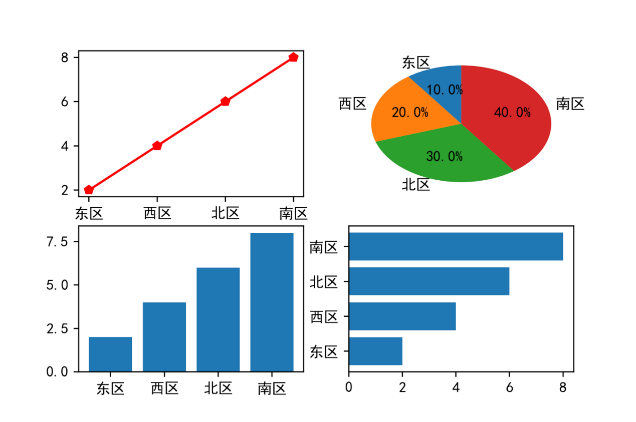图9-13
9.3.2　用pandas库绘图
Matplotlib库功能强大，但是相对底层。前面章节介绍过的数据分析库pandas，它的绘图功能就是基于Matplotlib库，这里继续介绍用Pandas库绘图的方法。
Series和DataFrame是Pandas库中主要的两种数据结构，都内置了plot方法，可以绘制图形。
1．Series.plot
Series是一个一维数据结构，它由index和value组成，类似于Excel表格中的一列数据，由行号和数据组成。根据这样一列数据，我们可以绘制各种图表，如柱状图、条形图、折线图、饼图等。
下面以实例说明。
⓿ import pandas as pd
import matplotlib.pyplot as plt
❶ plt.rcParams['font.sans-serif']=['SimHei']
❷ data=pd.Series([2,4,6,8],index=['1季度', '2季度', '3季度', '4季度'])
❸ fig=data.plot(kind='bar', title='2019年各季度销量(单位：万台)',
figsize=(20,16),fontsize=30)
❹ fig.axes.title.set_size(40)
plt.show()
语句⓿导入pandas库，并给它起别名pd，导入Matplotlib库的pyplot子模块，并给它起别名plt；语句❶是设置中文字体；语句❷构造数据；语句❸绘制柱状图并设置标题；语句❹设置标题字号，效果如图9-14所示。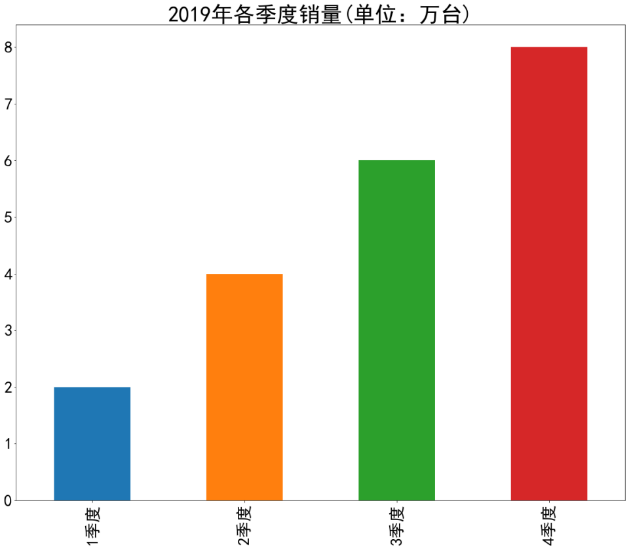图9-14
plot函数也有许多参数，例如参数kind控制图表类型，将kind='bar'修改为kind='pie'，图表将变为饼状图，效果如图9-15所示。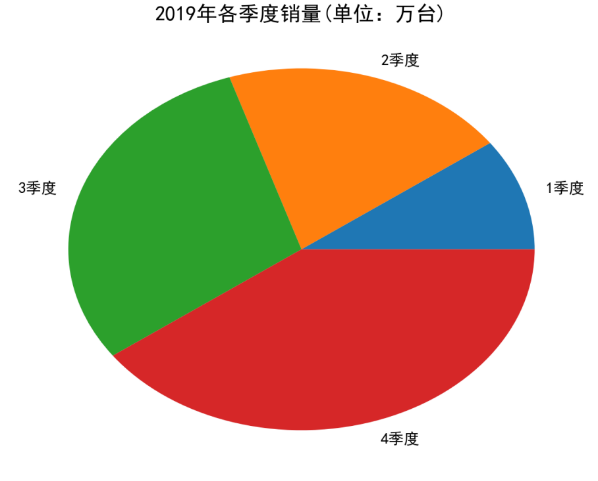图9-15
2．DataFrame.plot
DataFrame是一个二维结构，除了拥有index和value之外，还拥有column。它类似于一张Excel表格，由多行、多列构成。DataFrame由多个Series对象组成，无论是行还是列，单独拆分出来都是一个Series对象。
下面以实例说明。
   import pandas as pd
import matplotlib.pyplot as plt
plt.rcParams['font.sans-serif']=['SimHei']
⓿ data=pd.DataFrame([[3,8],[2,6],[6,1],[5,4]],
index=['1季度', '2季度', '3季度', '4季度'],columns=['手机', '电脑'])
❶ fig=data.plot(kind='bar',title='2019年各季度产品销量(单位：万台)',
figsize=(20,16),fontsize=30)
fig.axes.title.set_size(40)
plt.legend(loc='best',fontsize=30)
plt.show()
语句⓿构造了绘图所需数据；语句❶绘制图表，设置标题、图表区大小、字号；语句设置标题字号；语句设置图例字号，最后显示图表，效果如图9-16所示。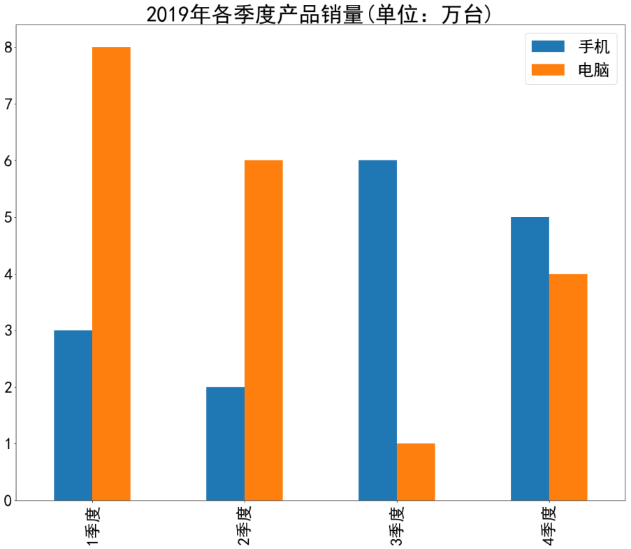图9-16
9.3.3　用Python绘制词云图
使用Python不仅可以绘制统计图表，还可以绘制各种可视化图，例如文本分析常用的词云图。

词云图，也叫“文字云”，是文本中出现频率较高的“关键词”的视觉化展现。频率越高，则字体越大、越突出，也越重要。相对于柱状图、折线图、饼图等用来显示数值数据的图表，词云图可以展示大量文本数据，通过过滤掉大量的低频低质的文本信息，使浏览者只要一眼扫过文本就可领略文本的主旨。

制作词云图要使用的库有：jieba、wordcloud、numpy、PIL、matplotlib。它们的安装方法都非常简单，直接用pip命令安装即可。
下面我们通过爬虫抓取电影《我们的未来》豆瓣点评，并制作点评文字的词云图。
⓿ import numpy as np
import jieba
from PIL import Image
from wordcloud import WordCloud, STOPWORDS
import matplotlib.pyplot as plt
❶ with open(r'H:\示例\第9章\影评.txt', 'rb') as f:
words=jieba.cut(text)
wordstr=' '.join(words)
wordcloud=WordCloud(font_path='H:\示例\第9章\msyh.ttf',
width=600, height=600, max_words=100, max_font_size=80,
stopwords=set(STOPWORDS),scale=4,background_color='white')
❹ wordcloud.generate(wordstr)
❺ wordcloud.to_file(r'H:\示例\第9章\wordcloud.png')
打开词云图，效果如图9-17所示（略）。
本文摘自《学Python，不加班——轻松实现办公自动化》这是一本关于如何利用Python提高日常办公效率的书，书中凝聚了作者多年的实践经验和独特思考，旨在帮助读者准确、高效地完成大量高重复度的工作。
本书汇集了日常办公和处理文档时常见的问题，通过实例的演示与讲解，帮助读者灵活有效地使用Python处理工作中遇到的问题。全书共11章，涵盖Python的各种应用场景，具体包括文件管理自动化，网络信息自动获取，TXT、XLS／XLSX、DOC／DOCX、PPT、PDF、图片文件的自动化处理，模拟鼠标、键盘操控本地软件，自动化运行管理等。本书力图淡化编程中的抽象概念，贴合工作场景，注重实战效果，通过对Python技术的巧妙讲解，帮助读者成为高效率的办公室“超人”。
展开全文Python 编程语言 程序员
• 说到数据可视化绘图，我们先想到的应该是matplotlib库，可以对其中的axes对象等调用不同的绘图方法（如axes.plot()）。 作为数据分析用的pandas库提供了Series DataFrame等类型的对象，我们也可以调用上述对象来绘图...python 数据分析 可视化 数据可视化
• 叫你如何使用python到如何使用numpy数组以及如何画图等用途，最后叫你如何使用sklearn框架调用机器学习方法
• 包含win32的matplotlib,pandas,dateutil,cycler,pytz,setuptools,pyparsing
• 用Python进行数据分析时，matplotlib和pandas是最常用到的两个库。 1、matplotlib库的应用 准备工作如下：打开ipython，输入命令分别导入numpy和matplotlib.pylab库。 [python] view plain copy ...numpy python 数据分析
• 转自：... Python之matplotlib模块安装 numpy 1、下载安装 源代码 http://sourceforge.net/projects/numpy/files/NumPy/ 安装 python2.7 setup.py install 2、测试 导入
• 1：以管理员身份打开anaconda prompt 2：查看当前环境 3：激活pytorch环境 4：安装命令 参考文章：https://blog.csdn.net/Qweiwei123/article/details/105154684pytorch 人工智能 python
• 在一幅图中绘制多个图案的方法很多，这阶段自学只要学会matplotlib和pandas模块中各自一种绘制图案即可。各自方法如下：（基于python语言编写） import matplotlib.pyplot as plt from matplotlib import gridspec...python子图绘制
• 基础概念环境 matplotlib 画图 numpy 处理数值型数组 pandas 处理数值型数组、字符串、时间序列、列表、字典等数据类型 一、综述 1、为什么要学习数据分析 有岗位需求 是Python数据科学的基础 是机器学习课程的...数据挖掘
• 1、查询查询默认pip版本 pip -V 如果出来打的结果是python3.x，需要安装python2下的pip。 2、为python2安装pip 如果出来打的结果是python3.x，为python2安装...4、具体安装PIL（Pillow）、opencv、numpy、matplotlib
• 前一篇文章讲述了数据预处理、Jieba分词文本聚类知识，这篇文章主要介绍Matplotlib和Pandas扩展包绘图的基础用法，同时引入Echarts技术，该技术主要应用于网站可视化展示中。本文内容以实例为主，给读者最直观的...可视化分析 Python Echarts
• 一、利用pandas进行数据分析 + matplotlib进行可视化展示 1.1 第一个绘图 （原本在matplotlib中需要几段代码，在pandas中只需要一行代码） import pandas as pd import numpy as np import matplotlib....
• 3.1、从文件中加载数据 3.2、从网页中加载数据 4.1、Series/DataFrame.plot()方法 4.2、参数详解 4.3、绘制多区域柱状图 4.4、绘制直方图 4.5、箱型图
• 基于numpy,matplotlib,pandas数学统计基础python库
• 画图在工作再所难免，尤其在做数据探索时候，下面总结了一些关于python画图的例子 ...# pylab 是 matplotlib 面向对象绘图库的一个接口。它的语法 Matlab 十分相近 import pandas as pd from ggplot
• Numpy、PandasMatplotlib三者的利弊分析 这三个库是使用python进行数据分析中最常用到的，numpy通常用来进行矢量化的计算，pandas通常用来处理结构化的数据，而matplotlib是用来绘制出直观的图表。 numpy底层是用...数据分析 python 机器学习 大数据 人工智能...